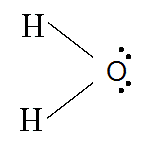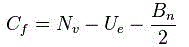# Lewis Structure

A Lewis structure is a structural representation of a molecule where dots are used to show electron position around the atoms and lines or dot pairs represent covalent bonds between atoms.

A way of representing atoms or molecules by showing electrons as dots surrounding the element symbol. One bond is represented as two electrons.

Lewis structures (also known as Lewis dot diagrams, electron dot diagrams, Lewis dot formulas, Lewis dot structures, andelectron dot structures) are diagrams that show the bonding between atoms of a molecule and the lone pairs of electrons that may exist in the molecule.

A Lewis structure can be drawn for any covalently bonded molecule, as well as coordination compounds. The Lewis structure was named after Gilbert N. Lewis, who introduced it in his 1916 article The Atom and the Molecule.

They are similar to electron dot diagrams in that the valence electrons in lone pairs are represented as dots, but they also contain lines to represent shared pairs in a chemical bond (single, double, triple, etc.)### Formal charge

In terms of Lewis structures, formal charge is used in the description, comparison, and assessment of likely topological and resonance structures by determining the apparent electronic charge of each atom within, based upon its electron dot structure, assuming exclusive covalency or non-polar bonding. It has uses in determining possible electron re-configuration when referring to reaction mechanisms, and often results in the same sign as the partial charge of the atom, with exceptions. In general, the formal charge of an atom can be calculated using the following formula, assuming non-standard definitions for the markup used:Where

Cf id the formal charge .

Nv represent the number of valence in a free atoms of the element .

Ue represent the number of unshared electron on the atoms .

Bn represent the total number of electrons in bond the atoms has with another .

The formal charge of an atom is computed as the difference between the number of valence electrons that a neutral atom would have and the number of electrons that belong to it in the Lewis structure. Electrons in covalent bonds are split equally between the atoms involved in the bond. The total of the formal charges on an ion should be equal to the charge on the ion, and the total of the formal charges on a neutral molecule should be equal to zero.